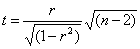# Statistics Assignment Help With T Test For Testing Significance

## 12.2.6 t test for testing significance of an observed sample correlation coefficient.If r is the observed correlation coefficient in a sample of n pairs of observations from a bivariate normal population, then Prof. Fisher proved that under the null hypothesis H0: ρ = 0, that is population correlation coefficient is zero, the statistic:Follows Student’s t – distribution with (n-2) degrees of freedom.

If the value of t comes out to be significant, we reject H0 at the level of significance adopted and conclude that ρ ≠ 0, that is, ‘r’ is significant of correlation in the population.

If t comes out to be non – significant then H0 may be accepted and we conclude that variables may be regarded as uncorrelated in the population.